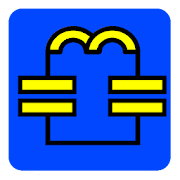# Electronic toolbox free

## Toolbox for electronic engineers hobbyists and students.

3.00 PRODUCTIVITY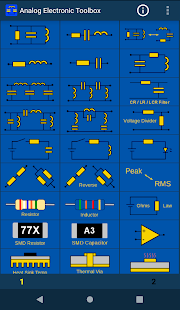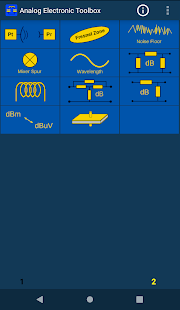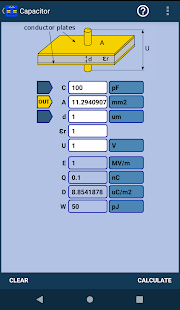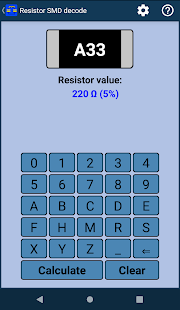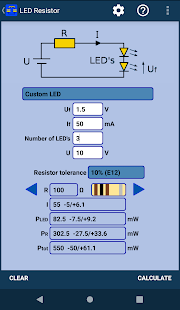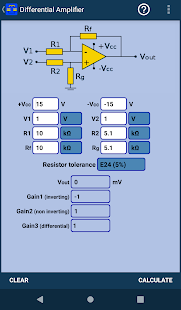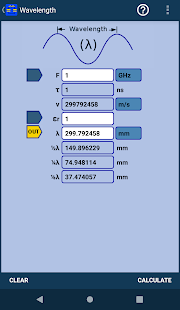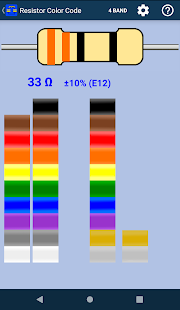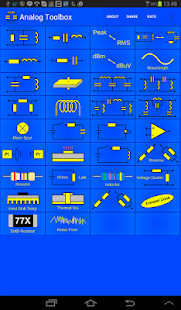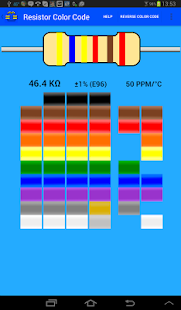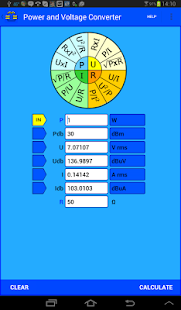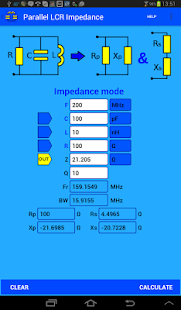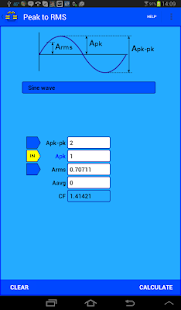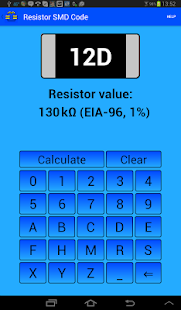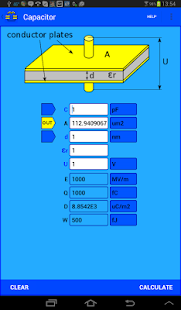Analog electronics toolbox for electronic engineers, hobbyists and students.

All the tools in this app are also included in the RF & Microwave Toolbox app:

LIKE and Share us:

Feature list:

1) PI, T and L attenuator
2) Power and voltage converter
3) Parallel LCR impedance/resonance
4) Series LCR impedance/resonance
5) Inductor impedance
6) Capacitance impedance
7) Ohm's Law
8) Radio equation calculator (1-way path loss)
9) Air Core Inductor Inductance Calculator
10) Capacitor impedance
11) Noise floor
12) Amplifier cascade (NF, Gain, P1db, OIP2, OIP3)
13) Low Pass filter
14) High Pass filter
15) Helical antenna
16) Band Pass filter
17) Band Stop filter
18) Peak to RMS (peak, RMS, average, CF)
19) Mixer harmonics
20) Resistor Color Code calculator.
21) PCB Trace Width and Clearance Calculator
22) Series and Parallel component (R, L and C) calculations.
23) Reverse Series and Parallel resistor calculations.
24) Inductor Color Code Calculator.
25) Capacitor Charge Calculator.
26) Led Resistor Calculator.
27) Voltage Divider Calculator.
28) Opamp Calculator
29) Wavelength calculator
30) LCR Parallel - Series Conversion Calculator
31) Inductor Charge Calculator.
32) Heat Sink temperature  calculator
33) Thermal via calculator
34) Resistor SMD Code calculator.
35) Bridged T Attenuator Calculator.
36) CR/LR/LCR Calculator (lowpass, highpass, bandpass and bandstop)

Added inductance,resistance, voltage drop, power dissipation, max and fuse current, rise and ambient temperature to the PCB via calculator (previous named Thermal via)

### Other versions

4.046 released on 05 July 2020 (522 days ago)

### Details and Recent Ratings

Size: 6 MB
Version: 4.050 by android-design.nl
Updated: 20 July 2020 (507 days ago)
Released: 23 October 2015
Installations: more than 10 000
5 Stars: 29
4 Stars: 29
3 Stars: 29
2 Stars: 29
1 Star: 29

### Similar apps

Android Game Categories
Android App Categories